# Development of Real-Time Fuzzy Synchronization of Chaos Based System for Image Encryption

Development of Real-Time Fuzzy Synchronization of Chaos Based System for Image Encryption
Electrical-Electronics Engineering Department, Engineering Faculty, Firat University, Elazig 23100, Turkey

Corresponding Author Email:
hasanguler@firat.edu.tr
Page:
1461-1467
|
DOI:
https://doi.org/10.18280/ts.380521
11 August 2021
|
Accepted:
12 October 2021
|
Published:
31 October 2021
| Citation

OPEN ACCESS

Abstract:

The concept of chaos, which has entered the literature since the 19th century, has been used for the protection of information with the development of information technologies in recent years. Chaos circuits are frequently used in secure communication systems because of its unpredictability and ease of modeling. In this paper, real-time fuzzy control method was used for master-slave synchronization of Rucklidge chaotic circuit. Also, sinusoidal signal and image encryption were used for application of secure communication process in LabVIEW platform. Data acquisition system was used to implement real time application. Fuzzy control performed synchronization at the end of the specified period and image encryption and decryption were successfully obtained in the developed system.

Keywords:

chaotic circuit, synchronization, fuzzy, image encryption

1. Introduction

With the spread of the Internet, it is observed that communication technologies over the web are increasing very rapidly. In addition, the process of transmitting a personal image to another receiver over the internet with the help of various applications takes place millions of times in a day. In addition to personal image transmission, many images including security and privacy are transmitted for military, medical and industrial applications. For this reason, it is an inevitable reality to create a secure communication platform. Many scientists are working hard to realize a more secure communication platform.

As a result of the first studies on image encryption, many standards such as advanced Encryption Standard (AES), Data Encryption Standard (DES), International Data Encryption Standard (IDES) and Linear Feedback Shift Register (LFSR) have been tried to be created, but the results obtained from them are large. It has been observed that the data transmission rate is low and it is insufficient in real-time applications [1-3]. As a solution to this, chaos-based studies are presented as a solution proposal.

Chaos theory is a method that is defined for explaining the tendency of parts of physical reality in a deterministic system. It is seen that behavior form of chaos arises in continuous, discrete and time-delayed systems. Chaotic behavior is used in different areas such as cryptology, driver circuits, increasing effectiveness of heuristic optimization methods, random number generators, secure communication systems, image processing and internet banking [4-17].

Chaos-based algorithms have been started to be developed for secure communication due to the fact that chaotic systems are extremely sensitive to initial conditions, have an ergodicity and complex structure, and a deterministic randomness structure.

The preferred methodology for chaos-based secure data transmission is to define two chaotic systems as Master-Slave and synchronize these two systems at a desired time. Chaos synchronization is a very important issue in the nonlinear system. Synchronization method is used to synchronize two identical chaotic systems according to different initial conditions. If Master-Slave form is designed for two chaotic systems, at time which is specified, the slave system will start following the master system and chaotic synchronization will be performed . In the secure communication systems, Master and Slave systems serve as transmitter and receiver respectively. Synchronization is required for secure communication to be successfully established between transmitter and receiver. Because of this reason, chaotic synchronization must be performed in real time.

When the studies published in the literature are examined, it is seen that many scientists have studied about chaotic system but there are few both the real time implementations of chaotic systems and the real time synchronization of chaotic systems [19-22].

Artificial intelligent methods such as Fuzzy system can be used for synchronization of switched chaotic systems for secure communications [23-25]. Fuzzy logic is frequently used in real-time applications, distinguishing itself from other artificial intelligence methods, due to the fact that it controls according to expert-based rule base and that the infrastructure of existing technological devices is suitable for real-time application. In this paper, the chaotic Rucklidge system’s Master-Slave synchronization for real-time image encryption and decryption applications was carried out using the fuzzy control method. In the realization of these transactions, LabVIEW is preferred for real-time applications due to its usage advantage and programming convenience by using DAQ (Data Acqusition) card, myRIO and cRIO within it in control, communication, biomedical systems [26-28].

Along with this introduction which defines that the aim of this study is to realize real-time artificial intelligence-based synchronization in an image encryption application for a secure communication, Rucklidge chaotic system examined in the paper is explained in the next section. The master-slave synchronization obtained between chaotic circuits via fuzzy controller and the used formulations are given in same section. The results of both simulation and real time synchronization outputs are given in Section 3. Also, secure communication and image encryption/decryption application have been carried out in this section. In this section, it is emphasized that a secure communication is achieved thanks to the algorithm performed by examining the values of points such as correlation coefficient (CC), peak signal to noise ratio (PSNR) and structural similarity index (SSIM). Finally, the results obtained from Section 3 are concluded in Section 4.

2. Material and Methods

2.1 Rucklidge chaotic system

The Rucklidge chaotic system used frequently in fluid mechanics is modelled with a third-order set of non-linear differential equations performing chaotic outputs . System’s differential equations are shown in Eq. (1).

$\dot{x}=-M x+L y-y z$

$\dot{y}=x$

$\dot{z}=-z+y^{2}$      (1)

where, x, y, z are state variables while M and L are positive system parameters. State space diagrams on x-y, x-z and y-z of Rucklidge chaotic systems are shown in Figure 1 and that on x-y-z plain of the system is given Figure 2. M and L values are chosen as 2 and 6.7 while the initial parameters of the chaotic circuit which are x(0), y(0) and z(0) are chosen as 1, 0 and 4.5, respectively .

## 1.pnga

## 1b.png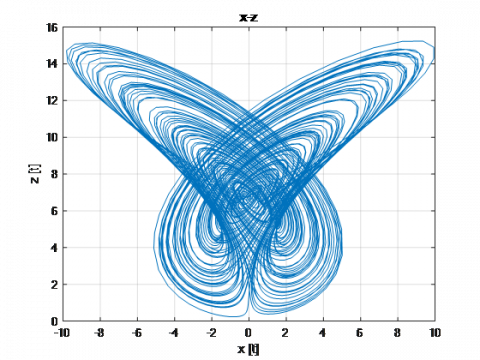b

## 1c.png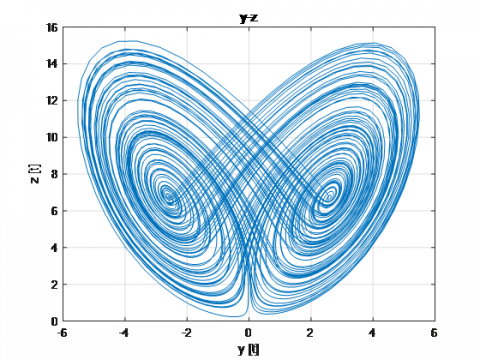c

Figure 1. Rucklidge chaotic system on a) x-y plain, b) x-z plain and c) y-z plain

## 2.png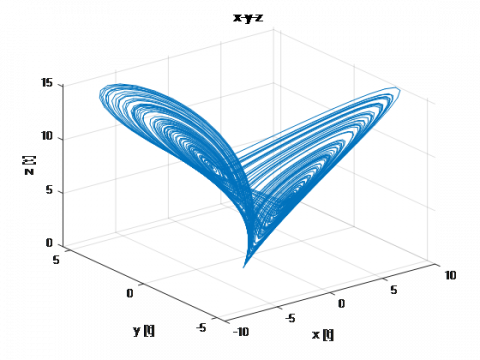Figure 2. Rucklidge chaotic system on x-y-z plain

2.2 Fuzzy synchronization of chaotic system

In the mid-1960s, Zadeh introduced Fuzzy set theory to deal with uncertainty and problems involving uncertainty. After the performance of fuzzy systems emerged, many scientists used this methodology to control their systems. Fuzzy systems are used in a wide range of fields such as control, biomedical, communication, mathematics and image processing. Fuzzy logic is successfully used in many applications in the world. Fuzzy system’s success is due to its suitability for both theoretical and practical application.

The fuzzy system architecture is given in Figure 3. There are four main parts in a fuzzy controller.

## 3.png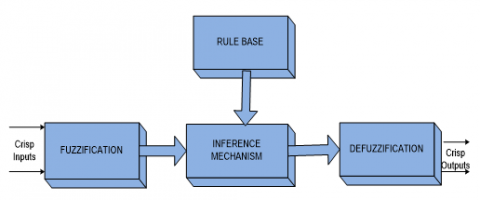Figure 3. Architecture of the fuzzy system

Error dynamic of chaotic system's each output for different initial conditions must be obtained to implement the chaotic synchronization by the fuzzy controller. Master system and Slave system’s differential equations consisting control function are illustrated in Eq. (2) and Eq. (3) .

$\dot{x}_{m}=-M x_{m}+L y_{m}-y_{m} Z_{m}$

$\dot{y}_{m}=x_{m}$

$\dot{z}_{m}=-z_{m}+y_{m}^{2}$     (2)

$\dot{x}_{s}=-M x_{s}+L y_{s}-y_{s} z_{s}+\mu_{1}(t)$

$\dot{y}_{s}=x_{s}+\mu_{2}(t)$

$\dot{z}_{s}=-z_{s}+y_{s}^{2}+\mu_{3}(t)$     (3)

where, μ1(t), μ2(t) and μ3(t) are the control functions. The errors of the system in Eqns. (2) and (3) are expressed in Eq. (4) as e1 = xsxm, e2 = ysymand e3 = zszm.

$\dot{e}_{1}=-M x_{s}+M x_{m}+L y_{s}-L y_{m}-y_{s} z_{s}+y_{m} z_{m}+\mu_{1}(t)$

$\dot{e}_{2}=x_{s}-x_{m}+\mu_{2}(t)$

$\dot{e_{3}}=-z_{s}+z_{m}+y_{s}^{2}-y_{m}^{2}+\mu_{3}(t)$     (4)

Master and Slave chaotic system’s initial conditions were chosen as in the study . To eliminate nonlinear components as the fuzzy control functions being, V1(t)=-k1e1, V2(t)=-k2e2, V3(t)=-k3e3.

$\mu_{1}(t)=y_{s} Z_{s}-y_{m} Z_{m}+V_{1}(t)$

$\mu_{2}(t)=V_{2}(t)$

$\mu_{3}(t)=y_{m}^{2}-y_{s}^{2}$     (5)

while calculating μ1(t), μ2(t) and μ3(t), k1, k2and k3 values are selected as k1=20, k2=30, k3=10, respectively.

Rucklidge system’s master-slave synchronization is illustrated in Figure 4.

## 4.png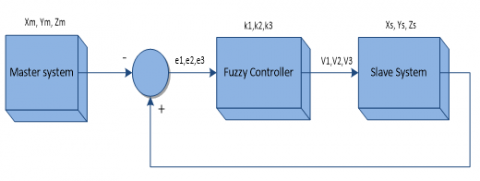Figure 4. The master-slave synchronization’s block diagram

The inputs of fuzzy system are error (e) and the nonlinear component signal (μt) for each state variable. Figure 5 shows the membership functions of the fuzzy system.

## 5.png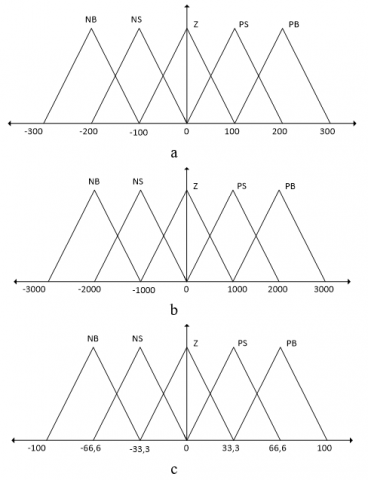Figure 5. a- The input MF for error, b- The input MF for nonlinear component signal and c- The output MF

Table 1 shows the rule table for the designed fuzzy control.

Table 1. Fuzzy controller’s rule table

 e µ NB NS Z PS PB NB NB NB NS NS Z NS NB NS NS Z Z Z NS Z Z PS PS PS Z Z PS PS PB PB Z PS PS PB PB

2.3 Image encryption on Master-Slave system

The block diagram to be used for image encryption in secure communication using the master-slave synchronization structure is shown in Figure 6 .

## 6.pngFigure 6. The schematic diagram of image encryption and decryption of chaos synchronization

2.4 Lyapunov exponents of the system

Lyapunov exponents mathematically indicate whether a system has time series. As it is known, since chaotic systems react very sensitively to initial conditions, this method expresses this sensitivity numerically. The fact that at least one of the Lyapunov exponents represented by L is positive indicates that the system is chaotic . L1=1.2528, L2=0.0018 and L3=-0.2546 are obtained when the parameters are fixed as specified (M=2 and L=6.7) and this system is started to run for initial conditions (1, 0, 4.5).

$D_{L}=j+\frac{\sum_{i}^{j} L_{i}}{L_{j+1}}=4,927$           (6)

As can be seen, because of the fact that one of the Lyapunov exponents in Figure 7 has a positive value, the necessary condition is fulfilled for being a chaotic system.

## 7.png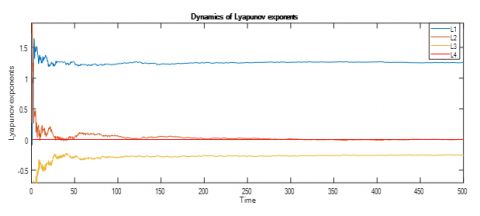Figure 7. Dynamics of Lyapunov exponent

3. Implementation of the System via LabVIEW Environment

In the paper, Rucklidge chaotic system’s simulation and real time synchronization is implemented in LabVIEW environment. Graphical code-based structure of master-slave synchronization using the equations of the chaotic system is given in Figure 8. Since there are 3 different state variables in the slave system, three different fuzzy controllers are created for each of them.

Before the implementation of image encryption, firstly, master-slave synchronization was observed with a sinusoidal signal of which amplitude is 0,1 V and frequency is 0,2 Hz. Results of simulation and real-time are shown in Figures 9 and 10. In both simulation and real time applications, the slave system of chaotic system with different starting conditions followed different trajectories until the 20th second, after this time, it followed master system.

## 8.png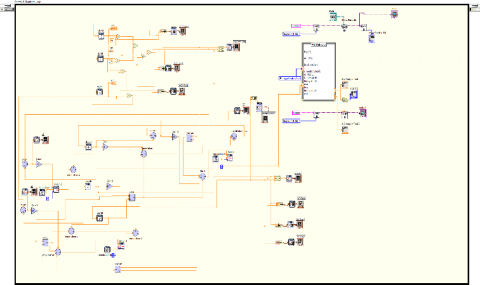Figure 8. Block diagram of Master-Slave system

## 9.png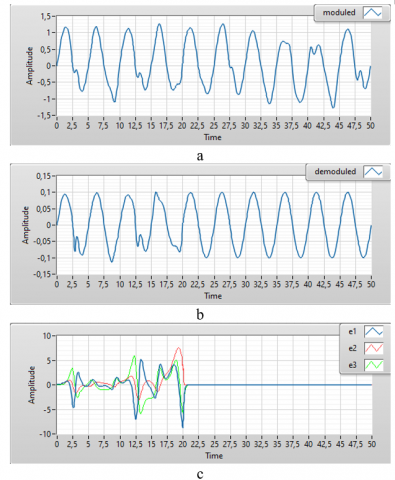Figure 9. a) The moduled signal, b) The demoduled signal c) the error of the state variables between Master and Slave systems

## 10.png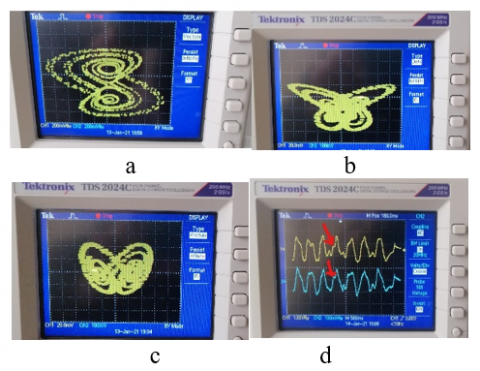Figure 10. The real time state-space diagrams of the chaotic Master Rucklidge System for a) x-y, b) x-z, c) y-z planes and d) The modulated signal (yellow) and the demodulated signal (blue)

## 11.png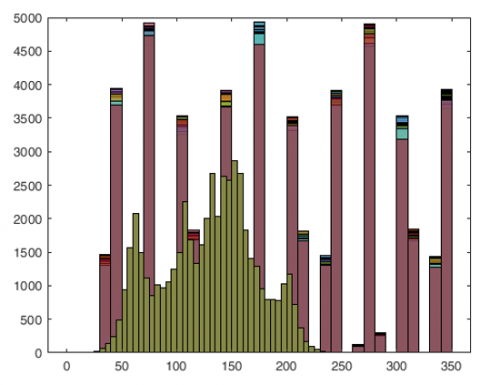Figure 11. The original image (green)-the encrypted image (red)

Real time results were obtained from NI-6009 data acquisition card (DAQ) which has 8 analogue inputs (14-bit, 48 kS/s), 2 analogue outputs (12-bit, 150 S/s) and 12 digital I/O, and 32-bit counter. Because of the fact that the voltage range of DAQ analogue outputs is between 0 and 5, the state variables need to be attenuated 20 times.

As can be seen in Figure 9-c, for each variable, after the fuzzy control was activated at 20 seconds, the slave system started to follow master system very quickly and the error between master and slave system quickly decreased to zero.

Three images were used for secure communication system. To see performance of the image encryption process, it was seen that there was almost no similarity between the two images according to the original and the encrypted images’ histograms. Histogram graph is only given for image-1 shown in Figure 11. Also, image encryption in front panel of LabVIEW platform and real-time results of three different images were shown in the Figures 12, 13, 14 and 15.

## 12.png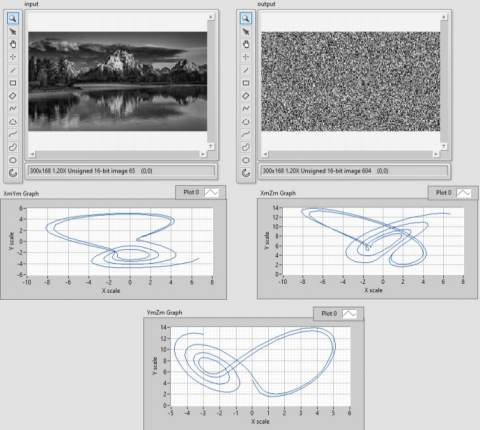Figure 12. Image encryption appearance of front panel

## 13.png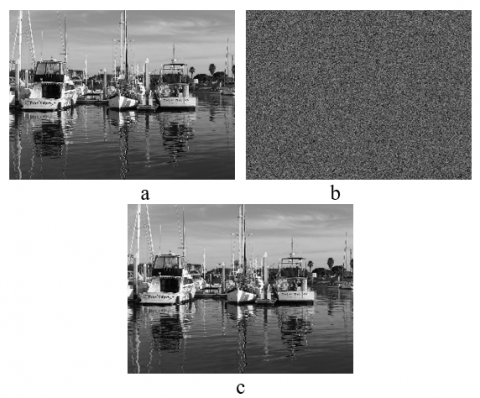Figure 13. a) Original grayscale image (U16) b) Encrypted image c) Decrypted image

## 14.pngFigure 14. a) Orginal grayscale image (U16) b) Encrypted image c) Decrypted image

## 15.pngFigure 15. a) Orginal grayscale image (U16) b) Encrypted image c) Decrypted image

Also, correlation coefficient (CC), peak signal to noise ratio (PSNR) and structural similarity index (SSIM) are examined for each three images in Table 1.

Table 2. Similarity parameters for the original and the encrypted image

 Image Dimension CC PSNR SSIM Image-1 1280*960 -0,0481 15,3261 0,03478 Image-2 300*138 0,0139 9,2598 0,02965 Image-3 228*221 -0,0515 13,0052 0,02611

The CC varies between -1 and 1. The similarity increases as the coefficient approaches 1, and when it approaches -1, the similarity decreases. Another structural similarity index is SSIM. If the value of it is approaching 1, the similarity of the two images increases, if it is approaching 0, the similarity of that decreases. Another calculated parameter is PSNR [30-33].

It can be said that according to the results obtained from the similarity parameters in Table 2, image encryption process is performed very successfully by using chaotic systems.

4. Conclusions

In literature, there are few studies about real time application on chaotic systems while there are many ones about simulation of it. In this paper, it was tried to implement real-time fuzzy synchronization and image encryption by using it for secure communication. A controller was designed to use Rucklidge chaotic system’s the Master-Slave synchronization with fuzzy control method. Simulation and real-time application were designed in LabVIEW platform. As can be seen from figures given above, after the controller is activated, 2 structures designed for the Rucklidge chaotic system follow each other.

By using DAQ card, it was tried to obtain real-time results on the designed chaotic system. Since the voltage limit of the DAQ card is between 0 and 5V, the signal sent to the output is attenuated in real time applications. Chaotic attractors and the modulated and the demodulated signals were obtained from an oscilloscope.

A secure communication simulation and image encryption implementation have been successfully tested with real-time fuzzy synchronization. Considering the degree of similarity calculated between the original images given as input to the system and the encrypted images, it is observed that the implemented system has yielded very successful results.

In future studies, it is aimed to realize different secure communication applications with the development of technological devices that can enable real-time applications of methodologies such as machine learning and deep learning.

References

 Khan, J.S., Ahmad, J. (2019). Chaos based efficient selective image encryption. Multidimensional Systems and Signal Processing, 30(2): 943-961. https://doi.org/10.1007/s11045-018-0589-x

 Schneier, B. (2007). Applied Cryptography: Protocols, Algorithms, and Source Code in C. New York: Wiley.

 Daemen, J., Rijmen, V. (2013). The Design of Rijndael: AES-The Advanced Encryption Standard. Berlin: Springer. https://doi.org/10.1007/978-3-662-60769-5

 Lorenz, E.N. (1963). Deterministic nonperiodic flow. Journal of Atmospheric Sciences, 20: 130-141. https://doi.org/10.1175/1520-0469(1963)

 Huang, J., Li, C., He, X. (2013). Stabilization of a memristor-based chaotic system by intermittent control and fuzzy processing. International Journal of Control, Automation and Systems, 11(3): 643-647. https://doi.org/10.1007/s12555-012-9323-x

 Chen, G., Ueta, T. (1999). YET another chaotic attractor. International Journal of Bifurcation and Chaos, 9(7): 1465-1466. https://doi.org/10.1142/S0218127499001024

 Feigenbaum, M.J. (1978). Quantitative universality for a class of non-linear Transformations. Journal of Statistical Physics, 19: 25-52. https://doi.org/10.1007/BF01020332

 Hénon, M. (1976). A two-dimensional mapping with a strange attractor. Communications in Mathematical Physics, 50(1): 69-77. https://doi.org/10.1007/BF01608556

 Mackey, M.C., Glass L. (1977). Oscillation and chaos in physiological control systems. Science, 197: 287-289. https://doi.org/10.1126/science.267326

 Uçar, A. (2002). A prototype model for chaos studies. International Journal of Engineering Science, 40: 251-258. https://doi.org/10.1016/S0020-7225(01)00060-X

 Özkaynak, F., Özer, A.B. (2010). A method for designing strong S-Boxes based on chaotic Lorenz system. Physics Letters A, 374(36): 3733-3738. https://doi.org/10.1016/j.physleta.2010.07.019

 Asker, M.E., Kürüm, H., Özer, A.B. (2015). Reduction of EMI by using chaotic sinusoidal PWM on vector controlled PMSM. International Journal of Scientific and Technological Research, 1(1): 83-93.

 Alatas, B., Akin, E., Özer, A.B. (2009). Chaos embedded particle swarm optimization algorithms. Chaos, Solitons & Fractals, 40(4): 1715-1734. https://doi.org/10.1016/j.chaos.2007.09.063

 Cuomo, K.M., Oppenheim, A.V., Strogatz, S.H. (1993). Synchronization of Lorenz-based chaotic circuits with applications to communications. IEEE Transactions on Circuits and Systems II: Analog and Digital Signal Processing, 40(10): 626-633. https://doi.org/10.1109/82.246163

 Yang, T., Chua, L.O. (1996). Secure communication via chaotic parameter modulation. IEEE Transactions on Circuits and Systems I: Fundamental Theory and Applications, 43(9): 817-819. https://doi.org/10.1109/81.536758

 Bulut, G.G., Catalbas, M.C., Guler, H. (2020). Chaotic systems based real-time implementation of visual cryptography using LabVIEW. Traitement du Signal, 34(4): 639-645. https://doi.org/10.18280/ts.370413

 Guler, H., Celik, V., Kaya, T., Erol, Y. (2018). The real time implementation of a chaotic system’s synchronization for secure communication. Tehnicki Vjesnik, 25: 43-48. https://doi.org/10.17559/TV-20160420113930

 Pecora, L.M. Carrol, T.L. (1990). Synchronization in chaotic systems. Physical Review Letters, 64: 821-824. https://doi.org/10.1103/PhysRevLett.64.821

 Sadoudi, S., Azzaz, M.S., Djeddou, M., Benssalah, M. (2009). An FPGA real-time implementation of the Chen’s chaotic system for securing chaotic communications. International Journal of Nonlinear Science, 7(4): 467-474.

 Koyuncu, I., Ozcerit, A.T., Pehlivan, I. (2014). Implementation of FPGA-based real time novel chaotic oscillator. Nonlinear Dynamics, 77: 49-59. https://doi.org/10.1007/s11071-014-1272-x

 Rodriguez-Bollain, A., Mata-Machuca, J.L., Martinez-Guerra, R. (2010). Synchronization of chaotic systems: A real-time application to Colpitts oscillator. CCE 2010 / Mexico, 60-65.

 Azzaz, M.S., Tanougast, C., Sadoudi, S., Bouridane, A., Dandache, A. (2010). An FPGA implementation of a feed-back chaotic synchronization for secure communications. CSNDSP 2010 / Newcastle, pp. 239-243.

 Kuo, C.L., Huang, L.C., Wang S.J., Lin J.S. (2013) Image encryption based on fuzzy synchronization of chaos systems. 2013 IEEE 37th Ann. Computer Software and Applications Conference, pp. 153-154. https://doi.org/10.1109/COMPSAC.2013.23

 Rucklidge, A.M. (1992). Chaos in models of double convection. Journal of Fluid Mechanics, 237: 209-229.

 Bulut, G.G., Guler, H. (2020). Fuzzy based chaotic synchronization of Chen systems. 1st Global Power, Energy and Communication Conference (IEEE GPECOM2019), pp. 30-34. https://doi.org/10.1109/GPECOM.2019.8778568

 Guler, H., Turkoglu, I., Ata, F. (2014). Designing intelligent mechanical ventilator and user interface using LabVIEW®. Arabian Journal for Science and Engineering, 39(6): 4805-4813. https://doi.org/10.1007/s13369-014-1090-y

 Guler, H., Ata, F. (2014). The comparison of manual and LabVIEW-based fuzzy control on mechanical ventilation. Proceedings of the Institution of Mechanical Engineers Part H-Journal of Engineering in Medicine, 228(9): 916-925. https://doi.org/10.1177/0954411914550513

 Poorna-chandra, B.R., Geevarghese, K.P., Gangadharan, K.V. (2014). Design and implementation of remote mechatronics laboratory for e-learning using LabVIEW and smartphone and Cross-Platform Communication Toolkit (SCCT). Procedia Technology, 14: 108-115. https://doi.org/10.1016/j.protcy.2014.08.015

 Sahin, M.E., Guler, H., Hamamci, S.E. (2020). Design and realization of a hyperchaotic memristive system for Communication System on FPGA. Traitement du Signal, 37(6): 939-953. https://doi.org/10.18280/ts.370607

 Catalbas, M.C., Gulten, A. (2018). A novel super resolution approach for computed tomography images by inverse distance weighting method. Journal of the Faculty of Engineering and Architecture of Gazi University, 33(2): 671-684. https://doi.org/10.17341/gazimmfd.416379

 Cai, Q.R. (2019). A secure image encryption algorithm based on composite chaos theory. Traitement du Signal, 36(1): 31-36. https://doi.org/10.18280/ts.360104

 Ma, K., Duanmu, Z., Yeganeh, H., Wang, Z. (2017). Multi-exposure image fusion by optimizing a structural similarity index. IEEE Transactions on Computational Imaging, 4(1): 60-72. https://doi.org/10.1109/TCI.2017.2786138

 Huang, Y., Niu, B., Guan, H., Zhang, S. (2019). Enhancing image watermarking with adaptive embedding parameter and PSNR guarantee. IEEE Transactions on Multimedia, 21(10): 2447-2460. https://doi.org/10.1109/TMM.2019.2907475